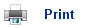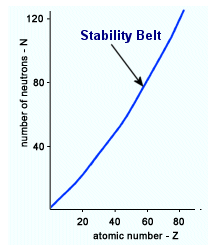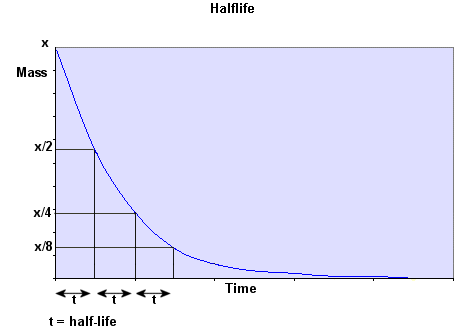# Ionising Particles

1. Some isotopes of elements are unstable. They break down spontaneously to produce ionising radiation and are described as radioactive.
2. Some isotopes give off radiation very quickly, for others the process takes thousands of years.
3. There are three different kinds of radiation alpha, beta and gamma.
4. All three types of radiation are capable of ionising atoms, so we refer to them as ionising radiation.

 Radiation Type of Radiation Mass (AMU) Charge Shielding material Alpha Particle 4 +2 Paper, skin, clothes Beta Particle 1/1836 -1 Plastic, glass, light metals Gamma Electromagnetic Wave 0 0 Dense metal, concrete, Earth

The atoms all want to be within the stable isotopes area, which is near to where n/p=1 for small particles and n/p=1.5 for larger ones. Radiation occurs in the isotopes to allow them to get into the stable area.## Nuclear Equations

1. Nuclear equations are used to summarise the processes which produce alpha and beta particles. They include the mass number, the atomic number, the charge and the chemical symbol for each particle involved.

## Alpha Decay

1. Alpha decay is most common in elements with a mass number that is larger than 83, The isotope produced from alpha decay will have a mass number four units lower and a nuclear charge that is 2 units lower.

23892U à 23490Th+ 42He

## Beta Decay

1. During beta decay, the mass number remains constant, but the proton number increases by one unit, because a neutron is converted into a proton and an electron.

146C à 147N + 0-1e

## Gamma Decay

1. Gamma decay is the emission of energy from the nucleus which is changing from a high energy level to a lower one. Gamma rays are a high frequency radiation.

## Half-lives

1. Unstable isotopes never stop emitting radiation.
2. Each different isotope decays at a different rate, and is not affected by temperature or pressure.
3. Radioactive decay depends on how much material there is; the amount of radiation is proportional to the mass of the element.
4. The half-life is unique to every isotope, and it is the amount of time it takes for half of the element to be decayed. The half life is always the same.## Useful books for revision:

Revise AS Chemistry for Salters (Written by experienced examiners and teachers of Salter's chemistry)Revise AS Chemistry for Salters (OCR) (Salters Advanced Chemistry)Home